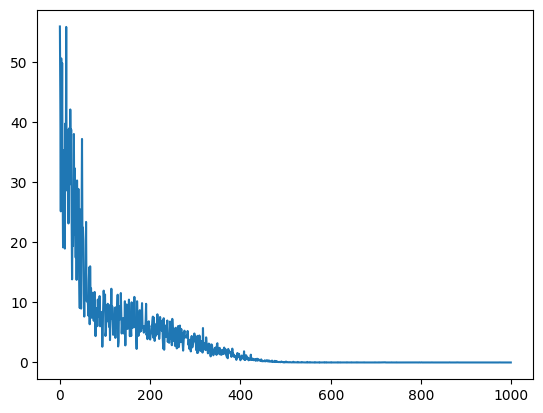# Simple regression problem - NN will not converge

I am training a neural network to predict the frequency of a sine wave. What happens is that the network learns to always predicts the mean value of the labels (the frequencies in the training data). What am I doing wrong?

Thanks!

The warning given:

``````/usr/local/lib/python3.10/dist-packages/torch/nn/modules/loss.py:536: UserWarning: Using a target size (torch.Size()) that is different to the input size (torch.Size([10, 1])). This will likely lead to incorrect results due to broadcasting. Please ensure they have the same size.
return F.mse_loss(input, target, reduction=self.reduction)
``````

is important here, as the MSE calculation will incorrectly broadcast `yhat - y_batch` to a shape of `[10, 10]` during the difference calculation before computing the mean. This broadcasting causes MSE to incorrectly exhibit the averaging effect observed as it would compute the “mean squared error” between each data point and all of the labels, not just the corresponding one.

By fixing the output shape with
`yhat = yhat.squeeze()`
I get

``````tensor(0.0034, grad_fn=<MseLossBackward0>)